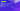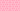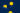Pricing# What is VaR (value at risk)?

When you’re evaluating your investments, it’s essential to understand the level of risk that each asset is faced with over a specific period. Traditionally, volatility was the primary method that firms used to assess risk, but VaR, or value at risk, has become more widely used over recent years. But what is VaR? Get the inside track on value at risk, including the benefits and limitations of this vital statistical technique.

## Meaning of VaR

Value at risk (VaR) is a financial metric that you can use to estimate the maximum risk of an investment over a specific period. In other words, the value at risk formula helps you to measure the total amount of potential losses that could happen in an investment portfolio, as well as the probability of that loss. Generally speaking, value at risk is used by investment or commercial banks to control the level of risk that their firm is exposed to via its investments.

## How do you calculate value at risk?

To calculate value at risk, you’ll need to assess the amount of potential loss, the probability of the occurrence of that loss, and the timeframe within which the loss may take place.

For example, your firm may determine that a particular asset has a 5% one-month VaR of 4%. This means that there’s a 5% chance that the value of the asset will decline in value by 4% during the one-month period that’s being assessed. Businesses can use a firm-wide value at risk assessment to determine the cumulative risks of the company’s aggregated investments, determining whether they have enough capital in place to cover any potential losses that may occur.

There are several value at risk formulas, or methods, that you may decide to use: the historical method, the parametric method, and the Monte Carlo method. Here’s a little more information about each of these value at risk formulas:

• Historical method – With the historical method, you’ll essentially re-organise real historical returns by ranking them from worst to best. For example, if you use market data from the past 100 days, the second-worst day will be 99% VaR, the third-worst day will be 98% VaR, and so on.

• Parametric method – Also referred to as the variance-covariance method, the parametric method assumes a normal distribution of returns. This means that you only need to estimate two factors, the expected return and the standard deviation, which allows you to plot a normal distribution curve. This value at risk formula is best suited to cases where you can reliably estimate the distributions.

• Monte Carlo method - With the Monte Carlo method, you can calculate value at risk using non-linear pricing models to randomly create different scenarios for future rates. Then, you can calculate VaR by estimating the change in value for each scenario and looking at the worst losses.

## What are the advantages of VaR finance?

There is a broad range of advantages associated with using value at risk to estimate risk. Firstly, it’s easy to understand, providing you with a single number to indicate a particular level of risk. It’s also applicable to a wide range of assets, including shares, bonds, currencies, and derivatives, which means that a broad range of institutions can use it. Finally, it’s important to remember that VaR finance is widely used, making it a good standard for buying or selling assets.

## Limitations of the value at risk formula

However, there are also a couple of limitations associated with VaR finance that you should understand. Most significantly, there’s no standardized protocol for determining asset/portfolio/firm-wide risk, and it’s possible that the statistics you use in your value at risk formula end up understating or magnifying the potential risks associated with your investment. It’s also important to note that the value at risk formula requires you to make assumptions, and if the assumptions aren’t correct, then the calculation won’t be either.

## We can help

GoCardless helps you automate payment collection, cutting down on the amount of admin your team needs to deal with when chasing invoices. Find out how GoCardless can help you with ad hoc payments or recurring payments.

Interested in automating the way you get paid? GoCardless can help

Contact salesSales

Contact sales

help@gocardless.com

Support

help@gocardless.com